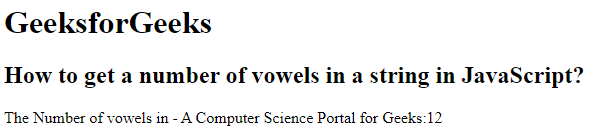Related Articles
How to get a number of vowels in a string in JavaScript?
• Last Updated : 18 Jan, 2021

The Approach of this article is to return the number of vowels in a string using Javascript. A vowel is also a letter that represents a sound produced in this way: The vowels in English are a, e, i, o, u.

Example:

```Input:GeeksForGeeks
Output:  5

Input: Hello Geeks
Output:    4```

Explanation: Here we create a user-defined function called “getvowels()” which reads a string and compare with list of vowels. It compares each character of a string with vowels. When the vowels is matches it will increment the value of the vowelsCount.

Example: Below code will illustrate the approach.

## HTML

 `<``html``>``    ``<``head``>``        ``<``title``>``            ``How to get a number of vowels``            ``in a string in JavaScript?``        ````    ````   ``<``body``>``       ``<``h1``>``           ``GeeksforGeeks``       ````       ``<``h2``>``           ``How to get a number of vowels in ``           ``a string in JavaScript?``       ````<``script``>``   ``function getVowels(string) {``      ``var Vowels = 'aAeEiIoOuU';``      ``var vowelsCount = 0;``      ``for(var i = 0; i < ``string.length` `; i++) {``         ``if (Vowels.indexOf(string[i]) !== -1) {``            ``vowelsCount += 1;``        ``}``      ``}``   ``return vowelsCount;``   ``}``   ``document.write("The Number of vowels in -"+``   ``" A Computer Science Portal for Geeks:" ``   ``+ getVowels("A Computer Science Portal for Geeks"));```````

Output:My Personal Notes arrow_drop_up